﻿ 基于光纤Bragg光栅的力传感技术研究 Research on Force Sensing Technology Based on Fiber Bragg Grating

Journal of Sensor Technology and Application
Vol. 07  No. 03 ( 2019 ), Article ID: 31207 , 9 pages
10.12677/JSTA.2019.73011

Research on Force Sensing Technology Based on Fiber Bragg Grating

Danping Jia, Can Cao, Hechi Ma

School of Information Science and Engineering, Shenyang University of Technology, Shenyang Liaoning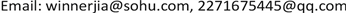Received: Jun. 18th, 2019; accepted: Jul. 3rd, 2019; published: Jul. 10th, 2019ABSTRACT

Based on fiber Bragg grating design force detection system to realize the robot’s perception of external forces, the principle of fiber grating force measurement is introduced. Elastic structure of sensor using the ANSYS finite element analysis for the static, modal and transient analysis to obtain the stress and strain of the elastic structure when subjected to forces/torques in all directions. The paste position of the fiber Bragg grating is determined. On the basis of the simulation, pressure experiments were carried out to determine the sensitivity, linearity, repeatability and stability of the force sensor.

Keywords:Fiber Bragg Grating, Force Sensor, Elastic Structure, Finite Element AnalysisCopyright © 2019 by author(s) and Hans Publishers Inc.

This work is licensed under the Creative Commons Attribution International License (CC BY).

http://creativecommons.org/licenses/by/4.0/1. 引言

2. 光纤Bragg光栅理论及传感原理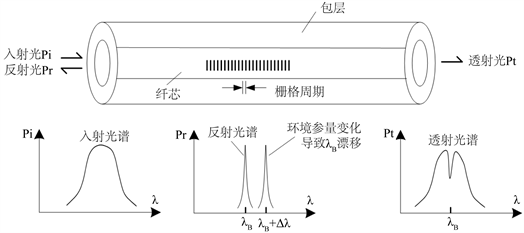Figure 1. Structure and schematic of FBG

${\lambda }_{B}=2{n}_{eff}*\Lambda$ (1)

${\Delta \lambda }_{B}/{\lambda }_{B}=\left(1-{P}_{\epsilon }\right)\cdot \epsilon$ (2)

3. 弹性体结构的仿真分析

ANSYS大型通用有限元分析软件是由美国ANSYS公司研发的，它可以对结构力学、热学、电磁场和声场、流体力学和耦合场等问题进行分析。本课题使用经典ANSYS中自带的建模模块来构建弹性体的模型  。在建模中，传感器的弹性梁及传感器的整体将通过布尔代数运算成为一个整体。使用的单元类型为SLOID187，弹性体的材料为304不锈钢，弹性模量为206 Gpa，泊松比为0.269，密度为7800 Kg/m3。采用手动方式进行网格划分，划分后得到90,110个节点，45,979个单元。然后进行静力学分析、模态分析和瞬态分析  。

3.1. 静力学分析

3.2. 模态分析Fx = 40N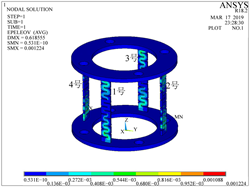Fy = 40NFz = 40N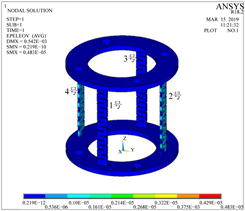Mx = 40N*mm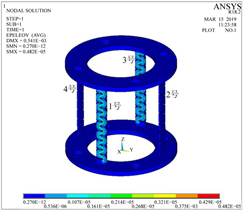My = 40N*mm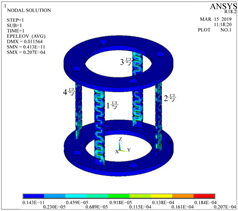Mz = 40N*mm

Figure 2. Stress cloud diagram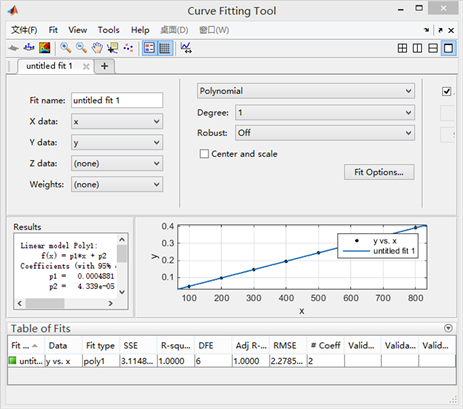Figure 3. Force-deformation fit straight line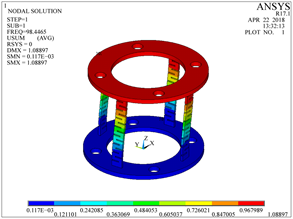一阶模态：98.447 Hz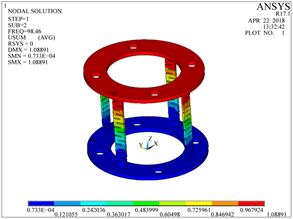二阶模态：98.460 Hz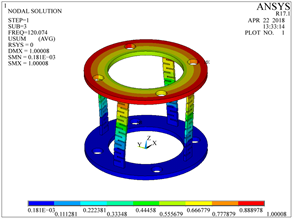三阶模态：120.07 Hz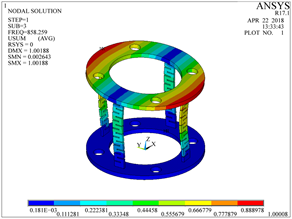四阶模态：858.26 Hz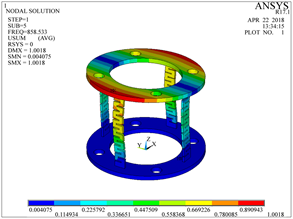五阶模态：858.53 Hz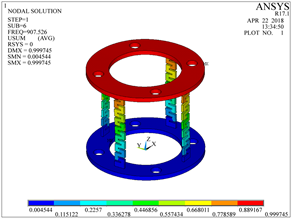六阶模态：907.53 Hz

Figure 4. Modal cloud mapTable 1. Elastomer natural frequency and mode shape

3.3. 瞬态分析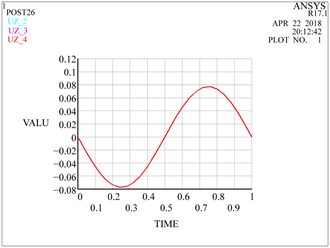正弦信号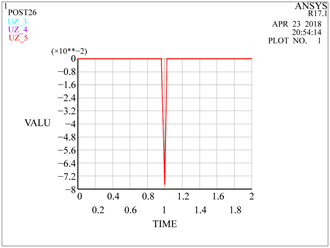脉冲信号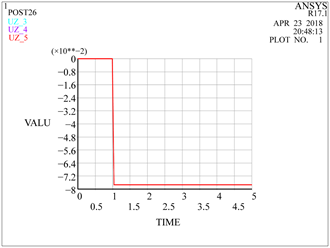阶跃信号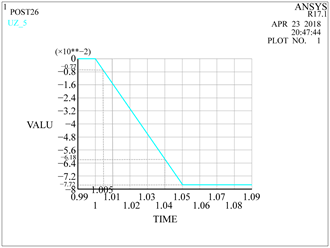阶跃延迟

Figure 5. Deformation of the elastic beam

4. 测力系统设计

5. 模拟实验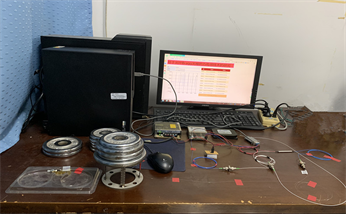Figure 6. Simulation experiment physical map

5.1. 弹性体结构Table 2. Basic geometric parameters of the elastomer (mm)Figure 7. Elastomer physical map

5.2. 灵敏度实验

$\Delta \lambda =0.0001617\ast F+0.04609\begin{array}{cc}& \end{array}{R}^{2}=0.9996$ (3)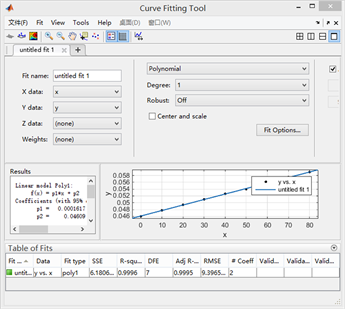Figure 8. Fit line diagram

5.3. 重复性实验Figure 9. Adding and unloading fit line graph

5.4. 稳定性实验Table 3. Measurements and errors of four times under different forces

6. 结论

Research on Force Sensing Technology Based on Fiber Bragg Grating[J]. 传感器技术与应用, 2019, 07(03): 95-103. https://doi.org/10.12677/JSTA.2019.73011

1. 1. Drake, S.H. and Watson, P.C. (1978) Method and Apparatus for Six Degree of Freedom Force Sensing. US Patent 4094192, 1978-6-13.

2. 2. Scheinman, V.D. (1969) Design of a Computer Controlled Manipulator. Stanford University, Computer Science Department, Stanford.

3. 3. Qiao, H., Dalay, B.S. and Parkin, R.M. (1993) Robotic Peg-Hole Insertion Operations Using a Six-Component Force Sensor. Proceedings of the Institution of Mechanical Engineers, Part C: Journal of Mechanical Engineering Science, 207, 289-306. https://doi.org/10.1243/PIME_PROC_1993_207_134_02

4. 4. 蒋奇, 宋金雪, 高芳芳, 李贻斌, 荣学文, 等. 基于光纤光栅的机器人多维力传感技术研究[J]. 光电子激光, 2014, 25(11): 2123-2129.

5. 5. 许会超, 苗新刚, 汪苏, 彭新改. 一种机器人多维光纤光栅力传感器[J]. 上海交通大学学报, 2016, 50(12): 1881-1884.

6. 6. 关寿华, 于清旭, 宋世德, 郑建洲. 长周期光纤光栅温度特性的理论与实验研究[J]. 传感技术学报, 2007, 20(3): 543-545.

7. 7. 刘涛, 杨凤鹏. 精通ANSYS [M]. 北京: 清华大学出版社, 2002: 1-517.

8. 8. Tu, Z.-F. (2010) ANSYS Finite Element Analysis Engineering Application Tutorial. China Building Industry Press, Beijing, 1-378.

9. 9. 刘正士, 陆益民, 陈晓东, 王国泰, 葛运建. 一种机器人多轴腕力传感器弹性体有限元分析[J]. 机械设计, 1998, 12(5): 12-14.